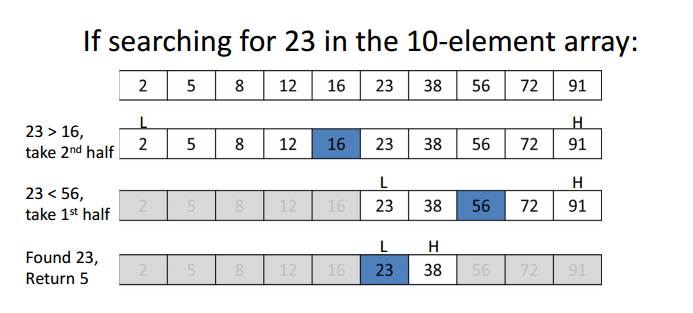# Binary Search

In computer science, binary search, also known as half-interval search, logarithmic search, or binary chop, is a search algorithm that finds the position of a target value within a sorted array.

Binary search compares the target value to the middle element of the array. If they are not equal, the half in which the target cannot lie is eliminated and the search continues on the remaining half, again taking the middle element to compare to the target value, and repeating this until the target value is found. If the search ends with the remaining half being empty, the target is not in the array.

Note: The array must be sortedPictorial representation of Binary Search Algorithm

Through the above algorithms, after each iteration, we are reducing the size of the searchable array by half, which leads to logarithmic time complexity.

# Unravelling Hidden Capabilities:

At the end, it’s all about optimization

Now, since you are aware of the basic implementation of Binary Search Algorithm, it’s time to move a step ahead and familiarise the with the powerful capabilities of Binary Search Algorithm.

Note: You can solve the below variations using other approaches as well, but here we’ll be using binary search, in order to understand it well and also optimise our code.

## Here is a list of the same:

• Searching in Reversed Sorted Array.
• Finding First and Last Occurance an Element.
• Finding Floor and Ceil of an Element.
• Finding the peak of an array.
• Finding Square Root of a number.
• Number of times a Sorted array is rotated.
• Searching in Nearly Sorted Array.
• Finding a position on an Element in an infinite array.
• Searching in Bitonic Array.
• Searching in 2-D Array.

In this article, I’ll be covering each variation in detail, with explanation and source code.

# 1. Searching in Reversed Sorted Array.

You are aware that the Binary Search Algorithm works on Sorted array. What if we reverse that array and come up with a reversed sorted array?

Approach:

Normally when the mid element is less than x, we search in the right section of the array and vice-versa, but here we will be considering an opposite approach. If the mid element is less than x, we search in the left section of the array and vice-versa.

# 2. Finding First and Last Occurance an Element.

Here we need to find the first and last occurrence of an element in an array. Let’s understand this with an example:

array = {1, 3, 10, 10, 10, 12, 13};

First Occurrence of 10 = 2

Last Occurrence of 10 = 4

Approach:

In this problem, we’ll search for x as we are searching normally. When we find the element, then there are two situations:

• To find the first Occurance, we keep searching for the element in the left part of the array.
• To find the last Occurance, we keep searching for the element in the right part of the array.

# 3. Finding Floor and Ceil of an Element.

## Floor of an Element

floor(x): Returns the largest integer that is smaller than or equal to x (i.e: rounds downs the nearest integer).

Approach:

Normally, we use == operator to check is mid is equal or not, this time we have to check if mid is ≤ that element. Also, we need to get close to that element, so even if we found an element which is less than mid, we’ll still move the left to mid+1 to get a better answer.

Code

## Ceil of an Element

ceil(x): Returns the smallest integer that is greater than or equal to x (i.e: rounds up the nearest integer).

Approach:

Normally, we use == operator to check is mid is equal or not, this time we have to check if mid is ≥ that element. Also, we need to get close to that element, so even if we found an element which is greater than mid, we’ll still move the right to mid-1 to get a better answer.

Code

# 4. Finding the peak of an array.

In this problem. you will not be provided with a sorted array, instead, you’ll be provided with an array which is monotonically increasing and monotonically decreasing in some parts.

Example:

a = {1, 4, 5, 10, 7, 3, 5, 2}

Peak = 10 at index 3

Observation:

• the first part (index 0 to 3) is strictly increasing and the second part (index 3 to 7) is strictly decreasing.
• the peak element is always greater than it’s neighbours
• No element other than the peak element is greater than both of its neighbours

We’ll consider the above observations to come up with an efficient solution

Code

Well, that’s it for today :(

I am sorry buddy, I really don’t want to leave it in between, but currently, I am a bit short on time, plus, if I cover all the ten variations in a single blog, it will be too lengthy, and lengthy blogs become boring :/

So yeah, that’s it for today, I will be back with the next six variations of Binary Search Algorithm in the next weekend and yes I can assure you that the second part is going to be much more interesting and we going to come up with something really cool, till then, enjoy, and thanks for reading till the end.

Happy Coding!

Feel free to reach out to me anytime if you want to discuss something. I would be more than happy if you send your feedback, suggestions.

Web: https://portfolio.abhisheksrivastava.me/
Instagram:
https://www.instagram.com/theprogrammedenthusiast/